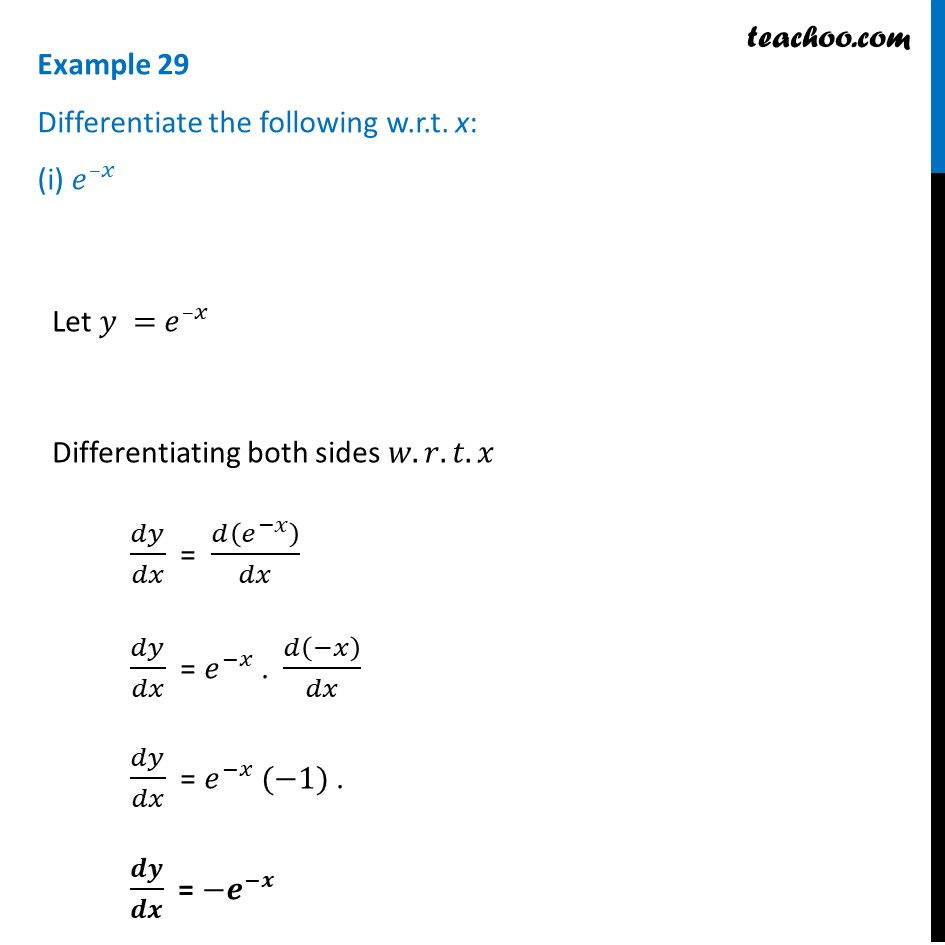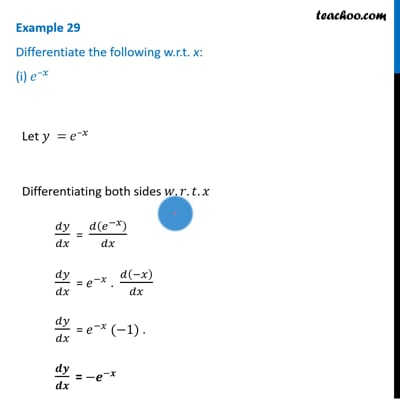Finding derivative of Exponential & logarithm functions

Chapter 5 Class 12 Continuity and Differentiability
Concept wiseThis video is only available for Teachoo black users

Introducing your new favourite teacher - Teachoo Black, at only ₹83 per month

### Transcript

Example 29 Differentiate the following w.r.t. x: (i) 𝑒^(–𝑥)Let 𝑦 = 𝑒^(–𝑥) Differentiating both sides 𝑤.𝑟.𝑡.𝑥 𝑑𝑦/𝑑𝑥 = 𝑑(𝑒^(−𝑥) )/𝑑𝑥 𝑑𝑦/𝑑𝑥 = 𝑒^(−𝑥) . 𝑑(−𝑥)/𝑑𝑥 𝑑𝑦/𝑑𝑥 = 𝑒^(−𝑥) (−1) . 𝒅𝒚/𝒅𝒙 = 〖−𝒆〗^(−𝒙)